Courses

# NCERT Solutions - Wave Optics Class 12 Notes | EduRev

## Class 12 : NCERT Solutions - Wave Optics Class 12 Notes | EduRev

The document NCERT Solutions - Wave Optics Class 12 Notes | EduRev is a part of the Class 12 Course Physics For JEE.
All you need of Class 12 at this link: Class 12

NCERT QUESTION

(Wave Optics)

Ques 10.1:
Monochromatic light of wavelength 589 nm is incident from air on a water surface. What are the wavelength, frequency and speed of (a) reflected, and (b) refracted light? Refractive index of water is 1.33.
Ans: Wavelength of incident monochromatic light,
λ = 589 nm = 589 × 10−9 m
Speed of light in air, c = 3 × 108 m/s
Refractive index of water, μ = 1.33

(a) The ray will reflect back in the same medium as that of incident ray. Hence, the wavelength, speed, and frequency of the reflected ray will be the same as that of the incident ray.

Frequency of light is given by the relation,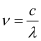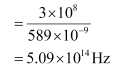Hence, the speed, frequency, and wavelength of the reflected light are 3 × 108 m/s, 5.09 ×1014 Hz, and 589 nm respectively.

(b) Frequency of light does not depend on the property of the medium in which it is travelling. Hence, the frequency of the refracted ray in water will be equal to the frequency of the incident or reflected light in air.

∴ Refracted frequency, ν = 5.09 ×1014 Hz

Speed of light in water is related to the refractive index of water as: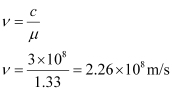Wavelength of light in water is given by the relation,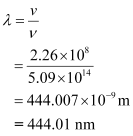Hence, the speed, frequency, and wavelength of refracted light are 2.26 ×108 m/s, 444.01nm, and 5.09 × 1014 Hz respectively.

Ques 10.2:
What is the shape of the wavefront in each of the following cases:
(a) Light diverging from a point source.
(b) Light emerging out of a convex lens when a point source is placed at its focus.
(c) The portion of the wavefront of light from a distant star intercepted by the Earth.
Ans: (a) The shape of the wavefront in case of a light diverging from a point source is spherical. The wavefront emanating from a point source is shown in the given figure.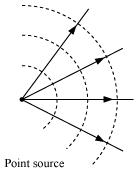(b) The shape of the wavefront in case of a light emerging out of a convex lens when a point source is placed at its focus is a parallel grid. This is shown in the given figure.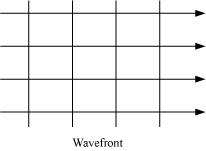(c) The portion of the wavefront of light from a distant star intercepted by the Earth is a plane.

Ques 10.3:
(a) The refractive index of glass is 1.5. What is the speed of light in glass? Speed of light in vacuum is 3.0 × 108 m s−1)
(b) Is the speed of light in glass independent of the colour of light? If not, which of the two colours red and violet travels slower in a glass prism?

Ans:  (a) Refractive index of glass, μ = 1.5
Speed of light, c = 3 × 108 m/s
Speed of light in glass is given by the relation,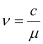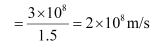Hence, the speed of light in glass is 2 × 108 m/s.

(b) The speed of light in glass is not independent of the colour of light.

The refractive index of a violet component of white light is greater than the refractive index of a red component. Hence, the speed of violet light is less than the speed of red light in glass. Hence, violet light travels slower than red light in a glass prism.

Ques 10.4:
In a Young’s double-slit experiment, the slits are separated by 0.28 mm and the screen is placed 1.4 m away. The distance between the central bright fringe and the fourth bright fringe is measured to be 1.2 cm. Determine the wavelength of light used in the experiment.

Ans:  Distance between the slits, d = 0.28 mm = 0.28 × 10−3 m
Distance between the slits and the screen, D = 1.4 m
Distance between the central fringe and the fourth (n = 4) fringe,
u = 1.2 cm = 1.2 × 10−2 m

In case of a constructive interference, we have the relation for the distance between the two fringes as: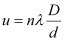Where,
n = Order of fringes = 4
λ = Wavelength of light used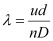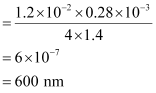Hence, the wavelength of the light is 600 nm.

Ques 10.5:
In Young’s double-slit experiment using monochromatic light of wavelengthλ, the intensity of light at a point on the screen where path difference is λ, is K units. What is the intensity of light at a point where path difference is λ /3
Ans:  Let I1 and I2 be the intensity of the two light waves. Their resultant intensities can be obtained as: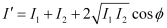Where,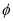= Phase difference between the two waves
For monochromatic light waves,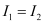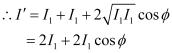Phase difference =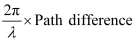Since path difference = λ,
Phase difference,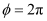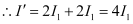Given,

I’ = K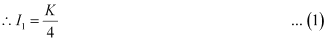When path difference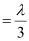,

Phase difference,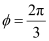Hence, resultant intensity,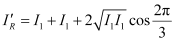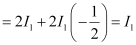Using equation (1), we can write: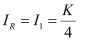Hence, the intensity of light at a point where the path difference is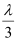is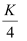units.

Ques 10.6:
A beam of light consisting of two wavelengths, 650 nm and 520 nm, is used to obtain interference fringes in a Young’s double-slit experiment.
(a) Find the distance of the third bright fringe on the screen from the central maximum for wavelength 650 nm.
(b) What is the least distance from the central maximum where the bright fringes due to both the wavelengths coincide?
Ans:  Wavelength of the light beam,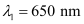Wavelength of another light beam,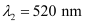Distance of the slits from the screen = D

Distance between the two slits = d

(a) Distance of the nth bright fringe on the screen from the central maximum is given by the relation,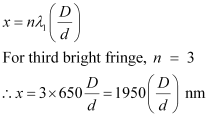(b) Let the nth bright fringe due to wavelength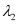and (n − 1)th bright fringe due to wavelength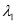coincide on the screen. We can equate the conditions for bright fringes as: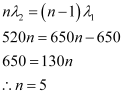Hence, the least distance from the central maximum can be obtained by the relation: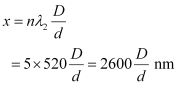Note: The value of d and D are not given in the question.

Ques 10.7:
In a double-slit experiment the angular width of a fringe is found to be 0.2° on a screen placed 1 m away. The wavelength of light used is 600 nm. What will be the angular width of the fringe if the entire experimental apparatus is immersed in water? Take refractive index of water to be 4/3.
Ans:  Distance of the screen from the slits, D = 1 m
Wavelength of light used,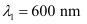Angular width of the fringe in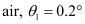Angular width of the fringe in water =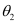Refractive index of water,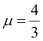Refractive index is related to angular width as: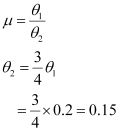Therefore, the angular width of the fringe in water will reduce to 0.15°.

Ques 10.8:
What is the Brewster angle for air to glass transition? (Refractive index of glass = 1.5.)
Ans:  Refractive index of glass,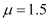Brewster angle = θ
Brewster angle is related to refractive index as: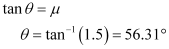Therefore, the Brewster angle for air to glass transition is 56.31°.

Ques 10.9:
Light of wavelength 5000 Å falls on a plane reflecting surface. What are the wavelength and frequency of the reflected light? For what angle of incidence is the reflected ray normal to the incident ray?

Ans:  Wavelength of incident light, λ = 5000 Å = 5000 × 10−10 m
Speed of light, c = 3 × 108 m
Frequency of incident light is given by the relation,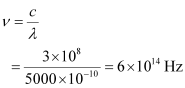The wavelength and frequency of incident light is the same as that of reflected ray. Hence, the wavelength of reflected light is 5000 Å and its frequency is 6 × 1014 Hz.

When reflected ray is normal to incident ray, the sum of the angle of incidence,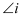and angle of reflection,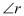is 90°.

According to the law of reflection, the angle of incidence is always equal to the angle of reflection. Hence, we can write the sum as: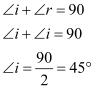Therefore, the angle of incidence for the given condition is 45°.

Ques 10.10:
Estimate the distance for which ray optics is good approximation for an aperture of 4 mm and wavelength 400 nm.
Ans:  Fresnel’s distance (ZF) is the distance for which the ray optics is a good approximation. It is given by the relation,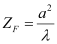Where,
Aperture width, a = 4 mm = 4 ×10−3 m
Wavelength of light, λ = 400 nm = 400 × 10−9 m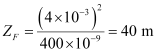Therefore, the distance for which the ray optics is a good approximation is 40 m.

Ques 10.11:
The 6563 Å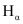line emitted by hydrogen in a star is found to be red shifted by 15 Å. Estimate the speed with which the star is receding from the Earth.
Ans:  Wavelength ofline emitted by hydrogen,
λ = 6563 Å
= 6563 × 10−10 m.
Star’s red-shift,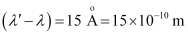Speed of light,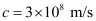Let the velocity of the star receding away from the Earth be v.
The red shift is related with velocity as: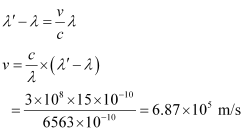Therefore, the speed with which the star is receding away from the Earth is 6.87 × 105 m/s.

Ques 10.12:
Explain how Corpuscular theory predicts the speed of light in a medium, say, water, to be greater than the speed of light in vacuum. Is the prediction confirmed by experimental determination of the speed of light in water? If not, which alternative picture of light is consistent with experiment?
Ans:  No; Wave theory
Newton’s corpuscular theory of light states that when light corpuscles strike the interface of two media from a rarer (air) to a denser (water) medium, the particles experience forces of attraction normal to the surface. Hence, the normal component of velocity increases while the component along the surface remains unchanged.

Hence, we can write the expression: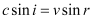… (i)

Where,

i = Angle of incidence
r = Angle of reflection
c = Velocity of light in air
v = Velocity of light in water
We have the relation for relative refractive index of water with respect to air as: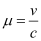Hence, equation (i) reduces to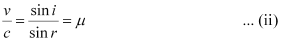But,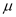> 1

Hence, it can be inferred from equation (ii) that vc. This is not possible since this prediction is opposite to the experimental results of cv.

The wave picture of light is consistent with the experimental results.

Ques 10.13:
You have learnt in the text how Huygens’ principle leads to the laws of reflection and refraction. Use the same principle to deduce directly that a point object placed in front of a plane mirror produces a virtual image whose distance from the mirror is equal to the object distance from the mirror.
Ans: Let an object at O be placed in front of a plane mirror MO’ at a distance r (as shown in the given figure).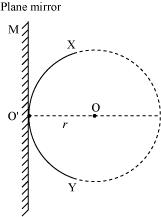A circle is drawn from the centre (O) such that it just touches the plane mirror at point O’. According to Huygens’ Principle, XY is the wavefront of incident light.

If the mirror is absent, then a similar wavefront X’Y’ (as XY) would form behind O’ at distance r (as shown in the given figure).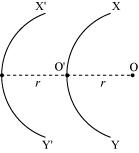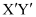can be considered as a virtual reflected ray for the plane mirror. Hence, a point object placed in front of the plane mirror produces a virtual image whose distance from the mirror is equal to the object distance (r).

Ques 10.14:
Let us list some of the factors, which could possibly influence the speed of wave propagation:
(i) Nature of the source.
(ii) Direction of propagation.
(iii) Motion of the source and/or observer.
(iv) Wave length.
(v) Intensity of the wave.

On which of these factors, if any, does
(a) The speed of light in vacuum,
(b) The speed of light in a medium (say, glass or water), depend?
Ans: (a) The speed of light in a vacuum i.e., 3 × 108 m/s (approximately) is a universal constant. It is not affected by the motion of the source, the observer, or both. Hence, the given factor does not affect the speed of light in a vacuum.

(b) Out of the listed factors, the speed of light in a medium depends on the wavelength of light in that medium.

Ques 10.15:
For sound waves, the Doppler formula for frequency shift differs slightly between the two situations:
(i) source at rest; observer moving, and
(ii) source moving; observer at rest.
The exact Doppler formulas for the case of light waves in vacuum are, however, strictly identical for these situations. Explain why this should be so. Would you expect the formulas to be strictly identical for the two situations in case of light travelling in a medium?

Ans: No
Sound waves can propagate only through a medium. The two given situations are not scientifically identical because the motion of an observer relative to a medium is different in the two situations. Hence, the Doppler formulas for the two situations cannot be the same.

In case of light waves, sound can travel in a vacuum. In a vacuum, the above two cases are identical because the speed of light is independent of the motion of the observer and the motion of the source. When light travels in a medium, the above two cases are not identical because the speed of light depends on the wavelength of the medium.

Ques 10.16:
In double-slit experiment using light of wavelength 600 nm, the angular width of a fringe formed on a distant screen is 0.1º. What is the spacing between the two slits?
Ans: Wavelength of light used, λ = 6000 nm = 600 × 10−9 m

Angular width of fringe,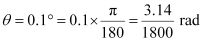Angular width of a fringe is related to slit spacing (d) as: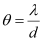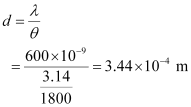Therefore, the spacing between the slits is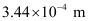.

Ques 10.17:
(a) In a single slit diffraction experiment, the width of the slit is made double the original width. How does this affect the size and intensity of the central diffraction band?
(b) In what way is diffraction from each slit related to the interference pattern in a double-slit experiment?
(c) When a tiny circular obstacle is placed in the path of light from a distant source, a bright spot is seen at the centre of the shadow of the obstacle. Explain why?
(d) Two students are separated by a 7 m partition wall in a room 10 m high. If both light and sound waves can bend around obstacles, how is it that the students are unable to see each other even though they can converse easily.
(e) Ray optics is based on the assumption that light travels in a straight line. Diffraction effects (observed when light propagates through small apertures/slits or around small obstacles) disprove this assumption. Yet the ray optics assumption is so commonly used in understanding location and several other properties of images in optical instruments. What is the justification?
Ans: (a) In a single slit diffraction experiment, if the width of the slit is made double the original width, then the size of the central diffraction band reduces to half and the intensity of the central diffraction band increases up to four times.

(b) The interference pattern in a double-slit experiment is modulated by diffraction from each slit. The pattern is the result of the interference of the diffracted wave from each slit.

(c) When a tiny circular obstacle is placed in the path of light from a distant source, a bright spot is seen at the centre of the shadow of the obstacle. This is because light waves are diffracted from the edge of the circular obstacle, which interferes constructively at the centre of the shadow. This constructive interference produces a bright spot.

(d) Bending of waves by obstacles by a large angle is possible when the size of the obstacle is comparable to the wavelength of the waves.

On the one hand, the wavelength of the light waves is too small in comparison to the size of the obstacle. Thus, the diffraction angle will be very small. Hence, the students are unable to see each other. On the other hand, the size of the wall is comparable to the wavelength of the sound waves. Thus, the bending of the waves takes place at a large angle. Hence, the students are able to hear each other.

(e) The justification is that in ordinary optical instruments, the size of the aperture involved is much larger than the wavelength of the light used.

Offer running on EduRev: Apply code STAYHOME200 to get INR 200 off on our premium plan EduRev Infinity!

## Physics For JEE

188 videos|517 docs|265 tests

,

,

,

,

,

,

,

,

,

,

,

,

,

,

,

,

,

,

,

,

,

;